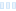Perturbation-style formulas depend on the #dz value remaining small enough
for fixed-point arithmetic. I managed to sabotage myself by setting a high
bailout value of 1e20 on this deep zoom with perturbation.

After reducing the bailout to 1e10 the formula worked beautifully.

(I wanted a high bailout because I was using smooth coloring)``````Disturbation_bad {
fractal:
credits="Andreas Hoppler;1/13/2023" antialiasing=yes
layer:
caption="Background" opacity=100 method=multipass
mapping:
center=-0.244850882604310407568430135/1.31143978352117385637300115
magn=2.5336664E16
formula:
maxiter=2000 filename="Standard.ufm" entry="Mandelbrot" p_start=0/0
p_power=3/0 p_bailout=1e20
inside:
transfer=linear filename="Standard.ucl" entry="Default"
outside:
transfer=linear filename="Standard.ucl" entry="Smooth" p_power=3/0
p_bailout=128.0
smooth=yes rotation=1 index=0 color=6555392 index=64 color=13331232
index=168 color=16777197 index=257 color=43775 index=343 color=512
opacity:
smooth=no index=0 opacity=255
}
``````
Perturbation-style formulas depend on the #dz value remaining small enough for fixed-point arithmetic. I managed to sabotage myself by setting a high bailout value of 1e20 on this deep zoom with perturbation. After reducing the bailout to 1e10 the formula worked beautifully. (I wanted a high bailout because I was using smooth coloring) ![63c1be8305b67.png](serve/attachment&amp;path=63c1be8305b67.png) ```` Disturbation_bad { fractal: title=&quot;Disturbation_bad&quot; width=1320 height=1241 layers=1 credits=&quot;Andreas Hoppler;1/13/2023&quot; antialiasing=yes layer: caption=&quot;Background&quot; opacity=100 method=multipass mapping: center=-0.244850882604310407568430135/1.31143978352117385637300115 magn=2.5336664E16 formula: maxiter=2000 filename=&quot;Standard.ufm&quot; entry=&quot;Mandelbrot&quot; p_start=0/0 p_power=3/0 p_bailout=1e20 inside: transfer=linear filename=&quot;Standard.ucl&quot; entry=&quot;Default&quot; outside: transfer=linear filename=&quot;Standard.ucl&quot; entry=&quot;Smooth&quot; p_power=3/0 p_bailout=128.0 gradient: smooth=yes rotation=1 index=0 color=6555392 index=64 color=13331232 index=168 color=16777197 index=257 color=43775 index=343 color=512 opacity: smooth=no index=0 opacity=255 } ````

0

That is definitely a good point!

That is definitely a good point!

Ultra Fractal author

0
25
views
1
replies
2
followers
live preview
Enter at least 10 characters.
WARNING: You mentioned %MENTIONS%, but they cannot see this message and will not be notifiedSaving...
Saved
All posts under this topic will be deleted ?
Pending draft ... Click to resume editing